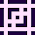## Aug 3, 2012

### Multiple Sequences Alignment in Python

In my previous post, I was created a funtion for align two single sequences. The following code will align several input sequences with several output sequences, based on their align score.

```#!/usr/bin/env python
# -*- coding: utf-8 -*-
#
# Recursive sequences alignment algorithm, based on LCS.
# Copyright (C) 2012  Gonzalo Exequiel Pedone
#
# This program is free software: you can redistribute it and/or modify
# the Free Software Foundation, either version 3 of the License, or
# (at your option) any later version.
#
# This program is distributed in the hope that it will be useful,
# but WITHOUT ANY WARRANTY; without even the implied warranty of
# MERCHANTABILITY or FITNESS FOR A PARTICULAR PURPOSE.  See the
# GNU General Public License for more details.
#
# You should have received a copy of the GNU General Public License
# along with This program. If not, see <http://www.gnu.org/licenses/>.
#
# Email   : hipersayan DOT x AT gmail DOT com
# Web-Site: http://hipersayanx.blogspot.com/
#
# This sequences alignment algorithm consist in findind the LCS of two sequences
# and then resolve the alignment of the sequences before and after the LCS
# recursively.

def unalignSequence(sequence=[]):
return [element for element in sequence if element != '']

def sequenceCount(sequences=[], sequence=[]):
count = 0

for s in sequences:
if unalignSequence(s) == unalignSequence(sequence):
count += 1

return count

def alignScore(aSequence1=[], aSequence2=[]):
score = 0

for i in range(len(aSequence1)):
if aSequence1[i] == aSequence2[i]:
# match
score += 1
else:
# gap
score -= 1

return score

def msa(sequences1=[], sequences2=[]):
sSequences1 = []
sSequences2 = []
scores = []

for sequence2 in sequences2:
for sequence1 in sequences1:
aSequence1, aSequence2 = alignSequences(sequence1, sequence2)
score = alignScore(aSequence1, aSequence2)

if scores == []:
sSequences1.append(aSequence1)
sSequences2.append(aSequence2)
scores.append(score)
else:
# Sort sequences by score.
imin = 0
imax = len(scores) - 1
imid = (imax + imin) >> 1

while True:
if score == scores[imid]:
sSequences1 = sSequences1[: imid + 1] + \
[aSequence1] + \
sSequences1[imid + 1:]

sSequences2 = sSequences2[: imid + 1] + \
[aSequence2] + \
sSequences2[imid + 1:]

scores = scores[: imid + 1] + \
[score] + \
scores[imid + 1:]

break
elif score < scores[imid]:
imax = imid
elif score > scores[imid]:
imin = imid

imid = (imax + imin) >> 1

if imid == imin or imid == imax:
if score < scores[imin]:
sSequences1 = sSequences1[: imin] + \
[aSequence1] + \
sSequences1[imin:]

sSequences2 = sSequences2[: imin] + \
[aSequence2] + \
sSequences2[imin:]

scores = scores[: imin] + [score] + scores[imin:]
elif score > scores[imax]:
sSequences1 = sSequences1[: imax + 1] + \
[aSequence1] + \
sSequences1[imax + 1:]

sSequences2 = sSequences2[: imax + 1] + \
[aSequence2] + \
sSequences2[imax + 1:]

scores = scores[: imax + 1] + \
[score] + \
scores[imax + 1:]
else:
sSequences1 = sSequences1[: imin + 1] + \
[aSequence1] + \
sSequences1[imin + 1:]

sSequences2 = sSequences2[: imin + 1] + \
[aSequence2] + \
sSequences2[imin + 1:]

scores = scores[: imin + 1] + \
[score] + \
scores[imin + 1:]

break

i = 0

while i < len(scores):
count1 = sequenceCount(sSequences1, sSequences1[i])
count2 = sequenceCount(sSequences2, sSequences2[i])

if count1 > 1 and count2 > 1:
del sSequences1[i]
del sSequences2[i]
del scores[i]

i -= 1
elif count1 > 1 and count2 < 2:
sSequences2[i] = unalignSequence(sSequences2[i])
sSequences1[i] = len(sSequences2[i]) * ['']
elif count1 < 2 and count2 > 1:
sSequences1[i] = unalignSequence(sSequences1[i])
sSequences2[i] = len(sSequences1[i]) * ['']

i += 1

return sSequences1, sSequences2

sequences1 = [list('ggagggcccagctcattcgggagaaagcct'),
list('tgtggggatcttgtggtgtgaac'),
list('gttgaaaagggtaccttcaattggaaccga'),
list('tcgggggtggccggaggacaacccagt'),
list('acttaaattatgagatc')]

sequences2 = [list('atattccctgtcagctctagttctttctcc'),
list('agctgccgggcgcg'),
list('gccacgagggaggcgggaggattca'),
list('ccctttcggttccaactatggtgcggggag')]

sSequences1, sSequences2 = msa(sequences1, sequences2)

for i in range(len(sSequences1)):
s1 = ''.join(['-' if element == '' else element \
for element in sSequences1[i]])
s2 = ''.join(['-' if element == '' else element \
for element in sSequences2[i]])

print(s1)
print(s2)
print()
```

1.This comment has been removed by the author.

2.Hi again. I definitively found this blog very helpful.

However, I suggest to change the input mode to the MSA function: It should be a single list array of sequences. Eg:
seqs = [CGAUGCAUGCAUGC, CAGUCGAUGCUAGC, CAGUCGAUGCA, ..., CAGUCGAUGCUA].
Also, in biology is very important to take into account that in MSA not all is insertion/deletion (gaps), but there are also substitutions. If you could implement the substitution, it could be greater! ;)

1.Hi, thank :)
When i wrote this post, i was working in a pipeline parser, a pipeline is a description on how a set of elements must be connected, my original idea was to write some kind of diff algorithm so when the pipeline description is changed the algorithm will minimize the creation/destruction/connection/diconnection of the elements.
As you said, this algorithm is an spinoff of the algorithm used in biology, but in this case all matches and gaps has the same weight, for that reason i just simplified the algorithm to this :P